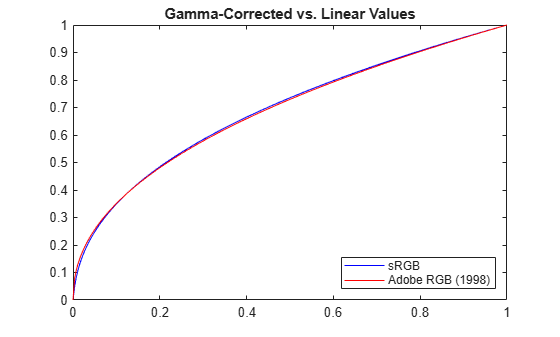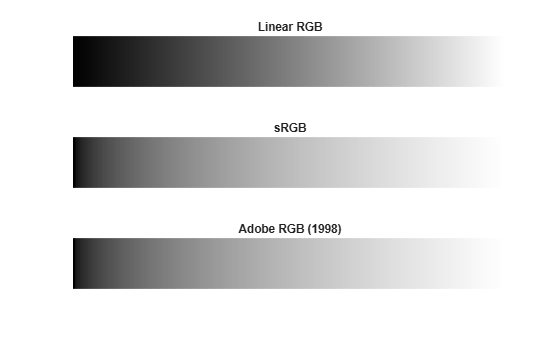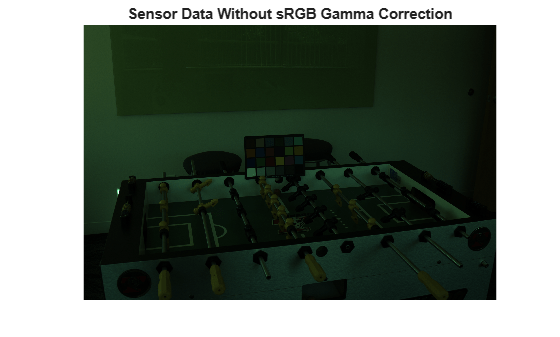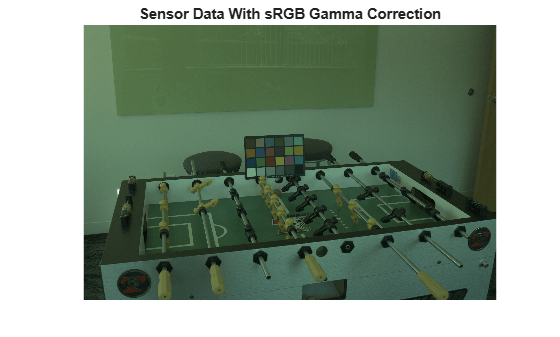lin2rgb

Apply gamma correction to linear RGB values

Description

example

B = lin2rgb(A) applies a gamma correction to the linear RGB values in image A so that B is in the sRGB color space, which is suitable for display.

example

B = lin2rgb(A,Name,Value) applies gamma correction using name-value pairs to control additional options.

Examples

collapse all

Define a range of linear values. This vector defines 257 equally spaced points between 0 and 1.

lin = linspace(0,1,257);

Apply gamma correction to the linear values based on the sRGB standard. Then apply gamma correction to the linear values based on the Adobe RGB (1998) standard.

sRGB = lin2rgb(lin);

Plot the gamma-corrected curves.

figure
title('Gamma-Corrected vs. Linear Values')For an alternative visualization, plot color bars representing each color space.

cb_lin = ones(30,257) .* lin;
cb_sRGB = ones(30,257) .* sRGB;

figure
subplot(3,1,1); imshow(cb_lin); title('Linear RGB')
subplot(3,1,2); imshow(cb_sRGB); title('sRGB');The gamma-corrected color spaces get brighter more quickly than the linear color space, as expected.

Open an image file containing minimally processed linear RGB intensities.

The image data is the raw sensor data after correcting the black level and scaling to 16 bits per pixel. Interpolate the intensities to reconstruct color by using the demosaic function. The color filter array pattern is RGGB.

A_demosaiced = demosaic(A,'rggb');

Display the image. To shrink the image so that it appears fully on the screen, set the optional initial magnification to a value less than 100.

figure
imshow(A_demosaiced,'InitialMagnification',25)
title('Sensor Data Without sRGB Gamma Correction')The image appears dark because it is in the linear RGB color space. Apply gamma correction to the image according to the sRGB standard, storing the values in double precision.

A_sRGB = lin2rgb(A_demosaiced,'OutputType','double');

Display the gamma-corrected image, setting the optional magnification.

figure
imshow(A_sRGB,'InitialMagnification',25)
title('Sensor Data With sRGB Gamma Correction');The gamma-corrected image looks brighter than the linear image, as expected.

Input Arguments

collapse all

Linear RGB color values, specified as a numeric array in one of the following formats.

• c-by-3 colormap. Each row specifies one RGB color value.

• m-by-n-by-3 image

• m-by-n-by-3-by-p stack of images

Data Types: single | double | uint8 | uint16

Name-Value Arguments

Specify optional comma-separated pairs of Name,Value arguments. Name is the argument name and Value is the corresponding value. Name must appear inside quotes. You can specify several name and value pair arguments in any order as Name1,Value1,...,NameN,ValueN.

Example: B = lin2rgb(I,'ColorSpace','adobe-rgb-1998') applies gamma correction to an image, I, according to the Adobe RGB (1998) standard.

Color space of the output image, specified as the comma-separated pair consisting of 'ColorSpace' and 'srgb' or 'adobe-rgb-1998'.

Data Types: char | string

Data type of the output RGB values, specified as the comma-separated pair consisting of 'OutputType' and 'double', 'single', 'uint8', or 'uint16'. By default, the output data type is the same as the data type of A.

Data Types: char | string

Output Arguments

collapse all

Gamma-corrected RGB image, returned as a numeric array of the same size as the input A.

Algorithms

collapse all

Gamma Correction Using the sRGB Standard

The gamma correction to transform linear RGB tristimulus values into sRGB tristimulus values is defined by the following parametric curve:

f(u) = -f(-u),             u < 0

f(u) = c ⋅ u,             0 ≤ u < d

f(u) = a ⋅ uɣ + b,      u ≥ d,

where u represents a color value with these parameters:

a = 1.055

b = –0.055

c = 12.92

d = 0.0031308

ɣ = 1/2.4

Gamma Correction Using the Adobe RGB (1998) Standard

The gamma correction to transform linear RGB tristimulus values into Adobe RGB (1998) tristimulus values uses a simple power function:

v = uɣ,           u ≥ 0

v = -(-u)ɣ,      u < 0,

with

ɣ = 1/2.19921875

 Ebner, Marc. "Gamma Correction." Color Constancy. Chichester, West Sussex: John Wiley & Sons, 2007.

 Adobe Systems Incorporated. "Inverting the color component transfer function." Adobe RGB (1998) Color Image Encoding. Section 4.3.5.2, May 2005, p.12.